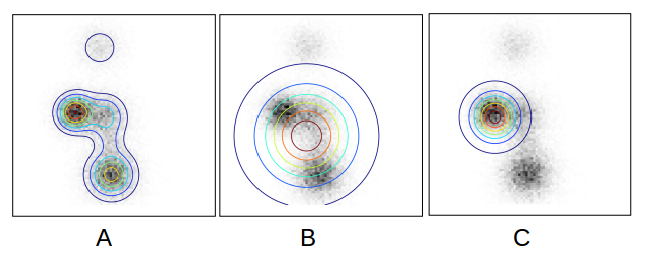# Maximum Likelihood and KL Divergence

Posted by GwanSiu on December 27, 2018

## 1. Maximum Likelihood

In this session, I will prove that maximum likelihood is equivalent to minimize KL divergence between true distribution $p_{data}$ and model distribution $p_{\theta}$.

suppose we have $N$ samples from $p_{data}$, i.e. $x\sim p_{data}$, then based on the law of large number, we have

thus the second term of $D_{KL}(p_{data}\Arrowvert p_{\theta})$ can be formulated

where NLL is negative log-lolikelihood, and c is constant.

## 3. Discussion on $D_{KL}(p_{\theta}\Arrowvert p_{data})$ and $D_{KL}(p_{data}\Arrowvert p_{\theta})$

$D_{KL}(p_{\theta}\Arrowvert p_{data})$ is defined and finite only if the support $p_{\theta}$ is contained in the support of $p_{data}$. The same to $D_{KL}(p_{data}\Arrowvert p_{\theta})$. The difference between $D_{KL}(p_{\theta}\Arrowvert p_{data})$ and $D_{KL}(p_{data}\Arrowvert p_{\theta})$:

1. Minimize $D_{KL}(p_{\theta}\Arrowvert p_{data})$ is to force the support of model distribution contains all example. it penalizes the model that assign a low probability mass to data sample, and likely finds a model $p_{\theta}$ that cover all modes of $p_{data}$, at the cost of placing probability mass where $p_{data}$ has none.

2. Minimize $D_{KL}(p_{\theta}\Arrowvert p_{data})$ ensure that the support of model distribution contains the support of empirical data distribution, which is a set of $p_{data}$. It penalizes model for generating implausible data, in other words, minimize $D_{KL}(p_{\theta}\Arrowvert p_{data})$ is mode searching, the optimal $p_{\theta}$ typically concentrate around the largest mode of $p_{data}$, at the cost of ignoring the smaller mode of $p_{data}$.

We assume we try to model a muitlmodal $P$ with simpler, unitmodel model $Q$, we show that figure A is the mutimodel $P$, and $B$ is the result of minimizing $D_{KL}(p_{\theta}\Arrowvert p_{data})$ and the output of minimizing $D_{KL}(p_{data}\Arrowvert p_{\theta})$.## Reference

 Huszár, Ferenc. “How (not) to train your generative model: Scheduled sampling, likelihood, adversary?.” arXiv preprint arXiv:1511.05101 (2015).

 Ke Li Jitendra Malik. “Implicit Maximum Likelihood Estimation” arXiv preprint arXiv:1809.09087v2 (2018).